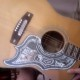### zine fodder

Symbols Used$\Bbb N$ the set of natural numbers, “en”$\Bbb Z$ the set of integers, “zee”$\Bbb Z^+$ the positive integers, “zee-plus”$\in$ “is an element of”; e.g.$-3\in \Bbb Z$,$-3\not\in \Bbb N$

# the number of elements for a (finite) set.
# (more generally) the “cardinality” of a set (finite or not)$X \cup Y$ the union of (sets) X and Y$X \cup Y$ the collection (set) of objects belonging X alone, or to Y alone, or to both.

W := C the symbol “W” stands for the code “C”
W := C “W equals C by definition

{…} the set containing “…” (typically a list of “elements”)$\{P(x)\}_{x\in S}$ the collection of all (objects) “x”, taken from the set “S”, such that the proposition “P(x)” is true$S^{\rightarrow}$ ess-arrow$S^{\rightarrow}$ the “successor” of S$\infty$ is well-known;
i’m lazy to look up aleph-null.

*********************************************************
the set of natural numbers, “en”
the set of integers, “zee”
the positive integers, “zee-plus”
“is an element of”; e.g. ,
# the number of elements for a (finite) set. # (more generally) the “cardinality” of a set (finite or not)
the union of (sets) X and Y;
the collection (set) of objects belonging
to X alone, or to Y alone, or to both.
W := C the symbol “W” stands for the code “C”
(“W equals C by definition“)
{…} the set containing “…” (typically a list of “elements”)
the collection of all (objects) “x”,
taken from the set “S”, such that
the proposition “P(x)” is true
the “successor” of S (“ess-arrow”)
is well-known; i’m lazy to look up aleph-null.

munging code is fun and easy.

1.vlorbik

getting actual hard copy is another story.

2.jd2718

So I tell the kids in a hushed voice that when I started taking more advanced classes, some of the language of mathematics changed….$\in “belongs to”; e.g. -3\in \Bbb Z, -3\not\in \Bbb N$

But now that I see the latex, I realize that my language might still be too school teacher-ish.

Oh, I also like “is different from.”

Jonathan

3.jd2718

lousy formula.$\in$ “belongs to”; e.g.$-3\in \Bbb Z$,$-3\not\in \Bbb N$

4.vlorbik

“belongs to” is good.
or even “is in”.

(“negative three is in the set of integers”…)

the LaTeX… and the plain vanilla TeX…
is $\in$ (and knuth is seldom wrong).

“is different from”
is sure as heck clearer english
than “is not equal to”…

i’ll go so far as to assert that this isn’t
(only) because we haven’t invoked the
“mathy” technical-feeling “equal sign”…
but because the concept “not”
is *hard*… probably at least
in part because it’s easy to *forget*…

5.vlorbik
6.jd2718

So off topic, but on. In logic, after years of tweaking, have decided that the words “invalid” and “weak” are to be strongly discouraged, forget what the book says. Opposite of valid is not valid. Opposite of strong is not strong. Let them chew on some nots.

jd

7.vlorbik

chewing on nots: good.
a set can be both open and closed.
what it *can’t* be is open and not open.

of valid/invalid
i defer to your years of tweaking.
it’s probably never occurred to me
*not* to use “invalid” as a synonym
for “not valid”. the temptation to
substitute “differently abled” is
easily suppressed…

somewhere down the hall somebody’s
making a very big deal of the fact that
“fail to reject” (the null hypothesis)
does *not* equate to “accept”.
you should never accept *anything*
based on a statistical argument
and textbooks make this explicit.
(it never does any good though.)

8.vlorbik

http://www.mathjax.org/

math on the web at last?

• ## (Partial) Contents Page

Vlorbik On Math Ed ('07—'09)
(a good place to start!)